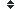graph (73 synonym found)

### Sort graph by:    Alphabetically| Length| Random | DefinitionDefinition of graph:

(n) : a diagram displaying data, in particular one showing the relationship between two or more variables; specifically, for a function $f\left(x_1, x_2, \ldots, x_n\right)$, the set of all tuples $\left(x_1, x_2, \ldots, x_n, f\left(x_1, x_2, \ldots, x_n\right)\right)$; an ordered pair $\left(V,E\right)$, where $V$ is a set of elements called vertices (or nodes) and $E$ is a set of pairs of elements of $V$, called edges; informally, a set of vertices together with a set edges that join these vertices
(v) : to draw a graph of (a function, data)
History
This word has been
viewed 4,785 times.

Related searches
for graph:

Most Recent

Most PopularDefinition of graph:

(n) : a diagram displaying data, in particular one showing the relationship between two or more variables; specifically, for a function $f\left(x_1, x_2, \ldots, x_n\right)$, the set of all tuples $\left(x_1, x_2, \ldots, x_n, f\left(x_1, x_2, \ldots, x_n\right)\right)$; an ordered pair $\left(V,E\right)$, where $V$ is a set of elements called vertices (or nodes) and $E$ is a set of pairs of elements of $V$, called edges; informally, a set of vertices together with a set edges that join these vertices
(v) : to draw a graph of (a function, data)